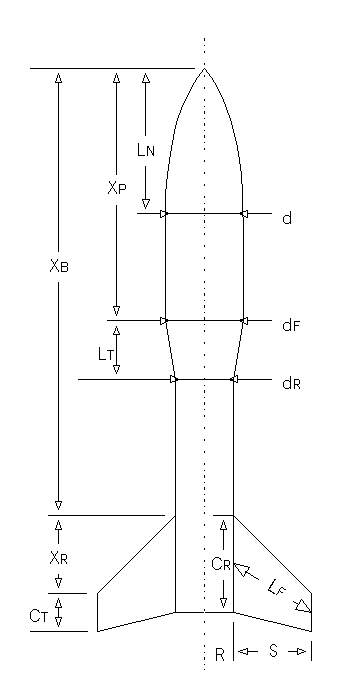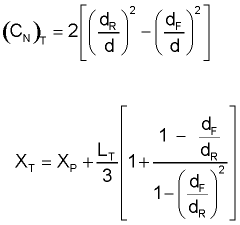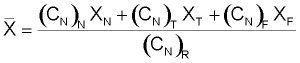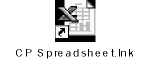Computing Center of Pressure

The Barrowman equations permit you to determine the stability of your rocket by finding the location of the center of pressure (CP). The value computed is the distance from the tip of the rocket's nose to the CP. In order for your rocket to be stable, you would like the CP to be aft of the center of gravity (CG).

The computation of CP isn't as hard as it looks at first. Check out the spreadsheet example at the bottom of this page.

You can find the CG of your rocket by simply finding the balance point after loading recovery system and motor. (Literally - balance the rocket on your hand - or finger - and that's the CG). You can then measure from the tip of the rocket's nose to the CG. The calculated CP distance should be greater than the measured CG distance by one rocket diameter. This is called "one caliber stability".

Terms in the equations are defined below (and in the diagram):

 LN = length of nose d = diameter at base of nose dF = diameter at front of transition dR = diameter at rear of transition LT = length of transition XP = distance from tip of nose to front of transition CR = fin root chord CT = fin tip chord S = fin semispan LF = length of fin mid-chord line R = radius of body at aft end XR = distance between fin root leading edge and fin tip leading edge parallel to body XB = distance from nose tip to fin root chord leading edge N = number of fins

Nose Cone Terms

(CN)N = 2
For Cone: XN = 0.666LN
For Ogive: XN = 0.466LN

Conical Transition TermsFin TermsFinding the Center of Pressure

Sum up coefficients: (CN)R = (CN)N + (CN)T + (CN)F

Find CP Distance from Nose Tip:Click for an example calculation (for my MinieMagg) using an Excel spreadsheet.

Who the Heck is Barrowman?

In March, 1967, James S. Barrowman of the National Aeronautics and Space Administration's Sounding Rocket Branch submitted a document entitled 'The Practical Calculation of the Aerodynamic Characteristics of Slender Finned Vehicles' as his Master's thesis to the School of Engineering and Architecture of the Catholic University of America. The document included, among other things, the simple algebraic method described above, capable of determining the center of pressure of a rocket flying subsonically and at small angles of attack to a high order of accuracy.

Questions

Your questions and comments regarding this page are welcome. You can e-mail Randy Culp (Tripoli #6926) for inquiries, suggestions, new ideas or just to chat.
Updated 24 August 2008